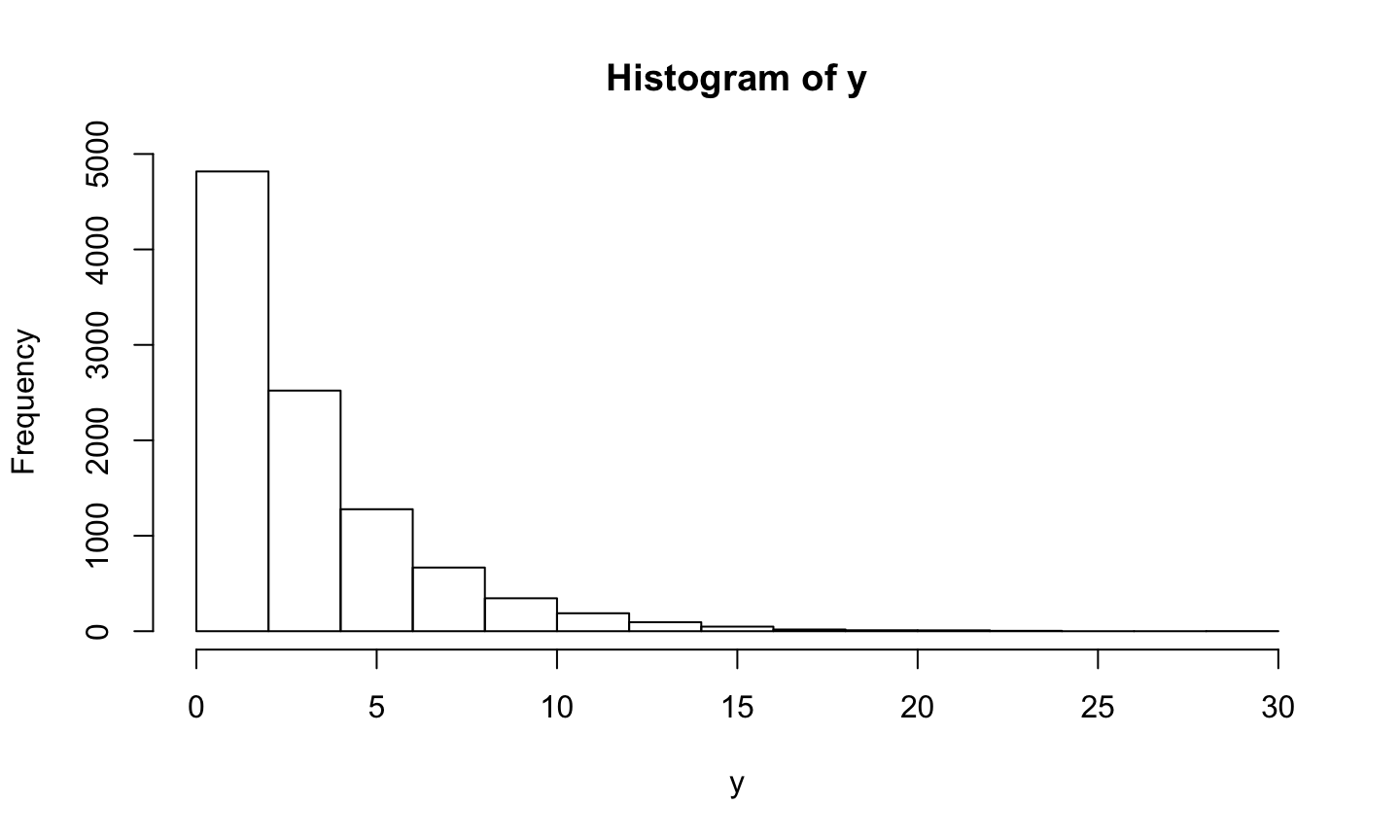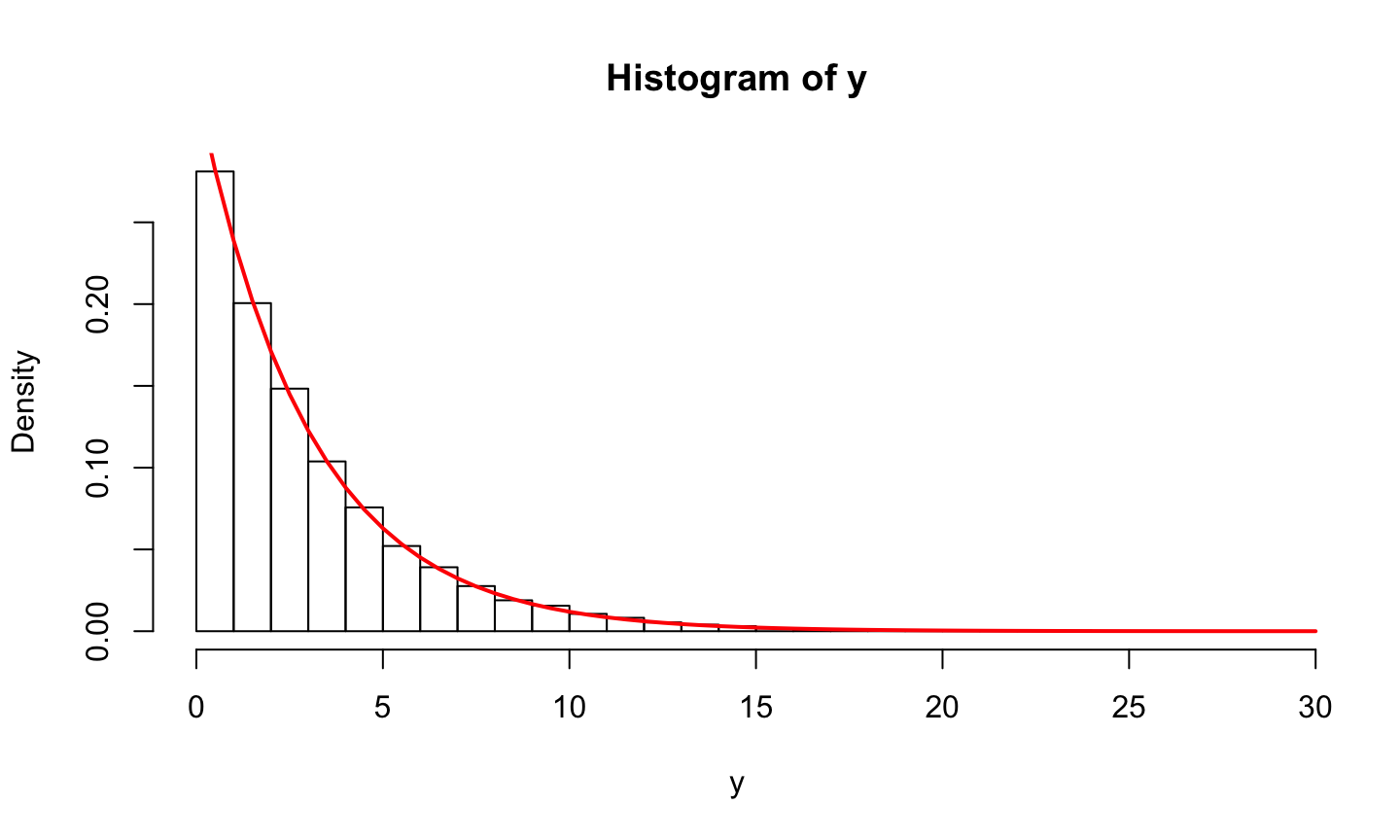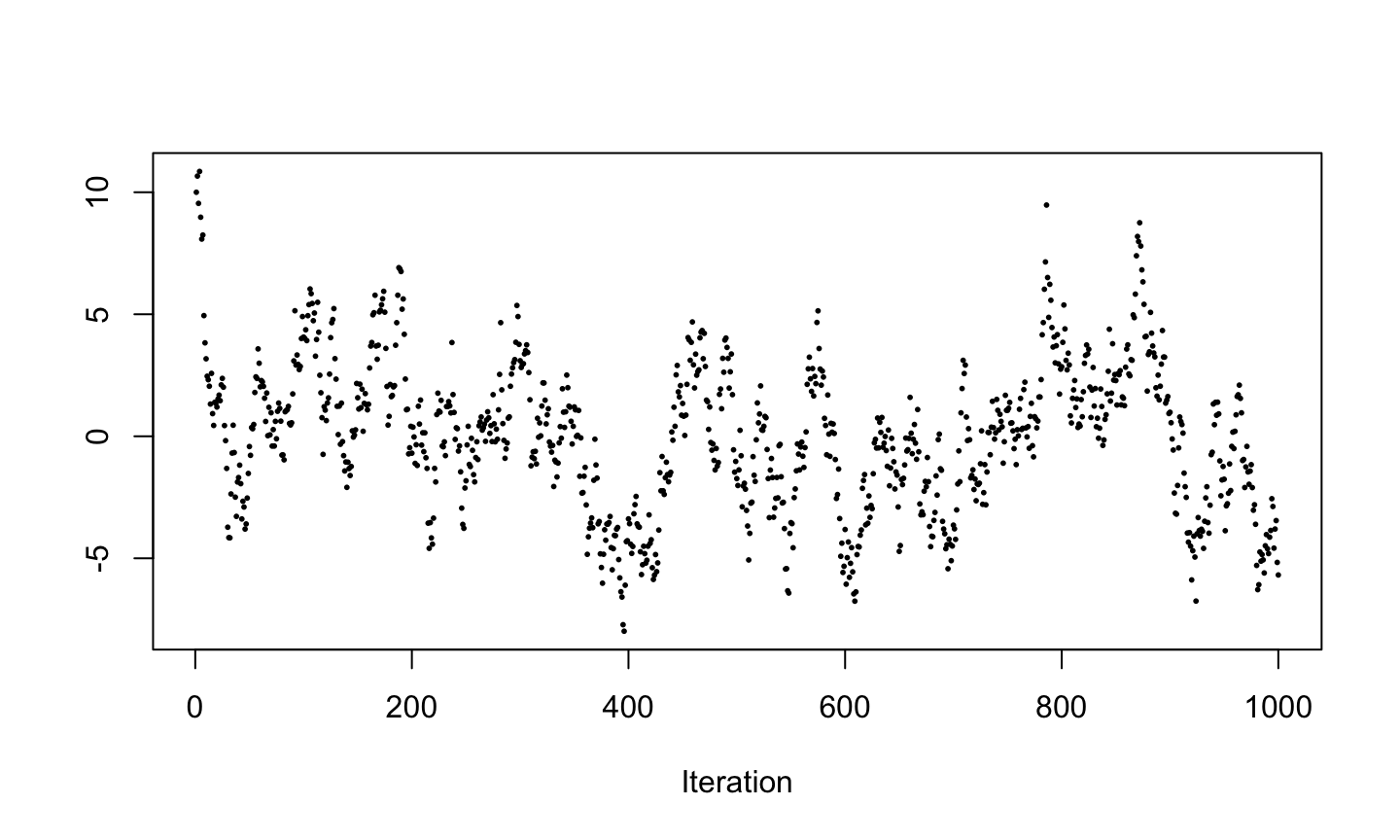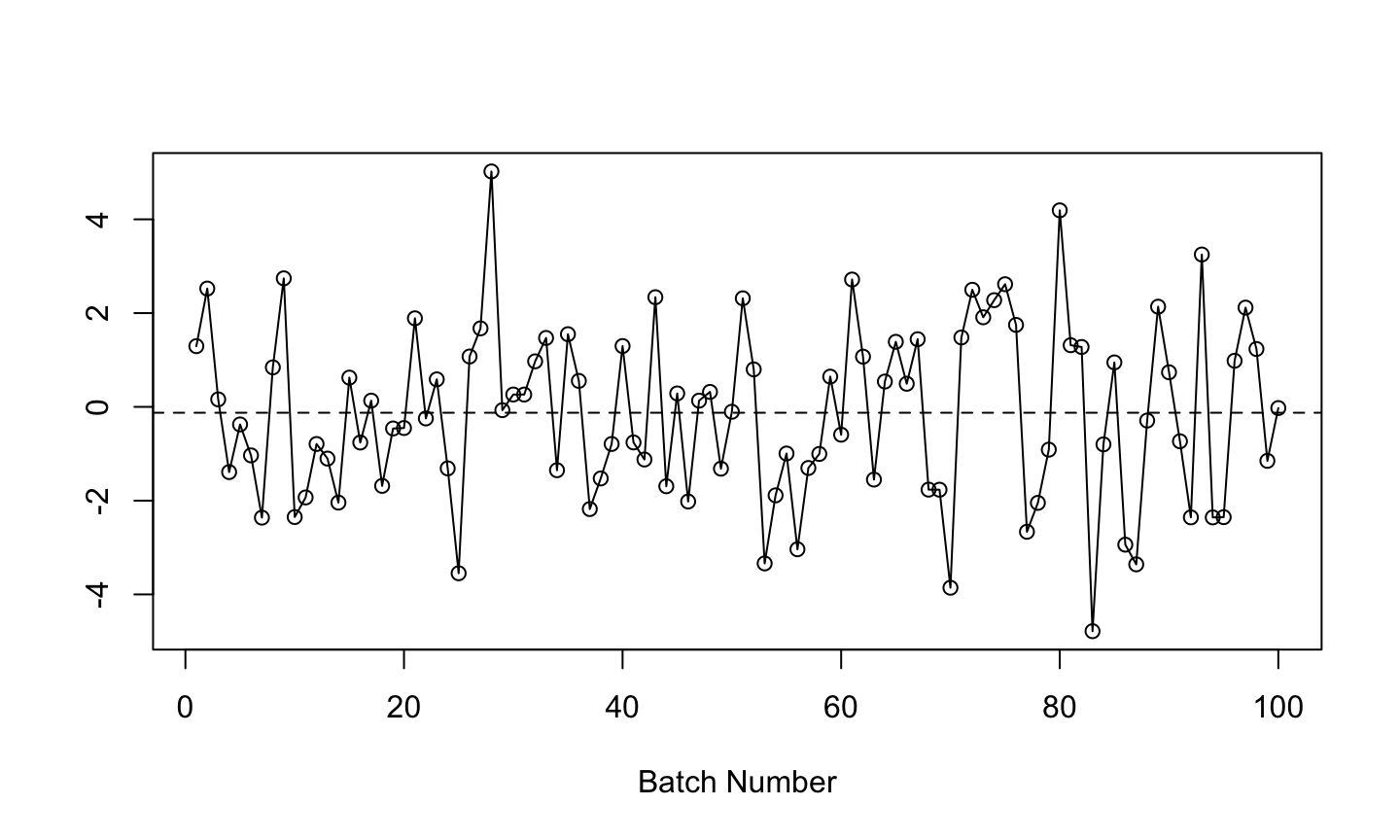## Agenda

• Simulations
• Monte Carlo
• Markov Chain Monte Carlo

## Simulations

• Historically, Bayesian methods were restricted by the need to perform integrations analytically.
• Modern Bayesian analysis typically performed by simulating the posterior distribution using Markov chain Monte Carlo (MCMC) methods.
• Prefer Monte Carlo methods to numerical integration because of their potential to deal with high-dimensional problems.
• Approximate Bayesian analysis using the analytic Laplace approximation and Monte Carlo methods.
• Prefer Monte Carlo methods to Laplace approximations in regression problems because when performing many predictions, only a single Monte Carlo sample is necessary to perform all predictions, whereas the Laplace method requires a separate analytic approximation for each prediction.

## Simulations

• Addressed simulations in STAT 206, where we discussed obtaining pseudorandom variates from a specified distribution.
• Quantile transform method, rejection sampling via accept-reject algorithm, simulating from mixtures, Box-Muller transformation, etc.

## Quantile transform method

Given $$U \sim \text{Uniform}(0,1)$$ and CDF $$F$$ from a continuous distribution. Then $$X = F^{-1}(U)$$ is a random variable with CDF $$F$$.

Proof: $P(X\le a) = P (F^{-1}(U) \le a) = P ( U \le F(a)) = F(a)$

• $$F^{-1}$$ is the quantile function
• If we can generate uniforms and calculate quantiles, we can generate non-uniforms
• Also known as the Probability Integral Transform Method

## Example: Exponential

Suppose $$X \sim \text{Exp}(\beta)$$. Then we have density $f(x) = \beta^{-1} e^{-x/\beta} I(0<x<\infty)$ and CDF $F(x) = 1 - e^{-x/\beta}$ Also $y = 1 - e^{-x/\beta} \text{ iff } -x/\beta = \log (1-y) \text{ iff } x = -\beta \log (1-y).$ Thus, $$F^{-1} (y) = -\beta \log(1-y)$$.

So if $$U \sim \text{Uniform}(0,1)$$, then $$F^{-1} (u) = -\beta \log(1-u) \sim \text{Exp}(\beta)$$.

## Example: Exponential

x <- runif(10000)
y <- - 3 * log(1-x)
hist(y)mean(y)
##  3.017044

## Example: Exponential

true.x <- seq(0,30, .5)
true.y <- dexp(true.x, 1/3)
hist(y, freq=F, breaks=30)
points(true.x, true.y, type="l", col="red", lw=2)## Box-Muller

• Box-Muller transformation transform generates pairs of independent, standard normally distributed random numbers, given a source of uniformly distributed random numbers
• Let $$U \sim \text{Uniform}(0,1)$$ and $$V \sim \text{Uniform}(0,1)$$ and set $R=\sqrt{-2\log U} \hspace{10mm} \textrm{ and } \hspace{10mm} \theta = 2\pi V$
• Then the following transformation yields two independent normal random variates $X=R\cos(\theta) \hspace{10mm} \textrm{ and } \hspace{10mm} Y=R\sin(\theta)$

## Rejection sampling

• The accept-reject algorithm is an indirect method of simulation
• Uses draws from a density $$f_y(y)$$ to get draws from $$f_x(x)$$
• Sampling from the wrong distribution and correcting it

## Rejection sampling

Theorem: Let $$X \sim f_x$$ and $$Y \sim f_y$$ where the two densities have common support. Define $M = \sup_{x} \frac{f_x(x)}{f_y(x)}.$ If $$M< \infty$$ then we can generate $$X \sim f_x$$ as follows,

1. Generate $$Y \sim f_y$$ and independently draw $$U \sim \text{Uniform}(0,1)$$
2. If $u < \frac{f_x(y)}{M f_y(y)}$ set $$X=Y$$; otherwise return to 1.

## Rejection sampling

• Recall $$M\ge 1$$ and $P(\text{STOP}) = B = P \left( u \le \frac{f_x(y)}{M f_y(y)} \right) = \frac{1}{M}.$
• Thus the number of iterations until the algorithm stops is Geometric($$1/M$$).
• Hence, the expected number of iterations until acceptance is $$M$$.

## Monte Carlo Integration

• Use these to approximate posterior expectations, posterior quantiles, or marginal posterior densities of interest.
• Focus will be on Monte Carlo and Markov chain Monte Carlo (MCMC) methods.

## Monte Carlo Integration

• Consider an integral that is an expectation, say $\mu = E\left\{ g(X) \right\} = \int g(x) f(x) dx$ where $$X$$ is a random variable with density $$f(x)$$. Assume this expectation actually exists, i.e. $$\mu < \infty$$.
• Only generally applicable tool for approximating integrals like this is so-called Monte Carlo integration.
• Suppose we can simulate an iid sequence $$X_1, X_2, \dots$$ of random variables having density $$f(x)$$. Then $Y_i = g(X_i), \quad i = 1,2,\dots$ is an iid sequence of random variables having mean $$\mu$$, which is the integral we want to evaluate.

## SLLN

• The SLLN says that if $$E|Y| < \infty$$ then with probability 1 as $$n \to \infty$$ $\bar{Y}_n = \frac{1}{n} \sum_{i=1}^n Y_i \to \mu .$
• We can approximate $$\mu$$ with an arbitrary level of precision if we only average over a sufficiently large number of simulations.
• Using $$\bar{Y}_n$$ as an approximation for $$\mu$$ is the “Monte Carlo method.” We saw multiple examples of this last quarter.

## Example

• Suppose $$X_1, X_2, \dots, X_m$$ are a random sample from a standard normal distribution. What is the relative efficiency of the sample mean compared to a 25% trimmed mean $$\hat{\theta}$$ as an estimator of the true unknown population mean $$\theta$$?
set.seed(1)
mdat <- 30 # data sample size
nsim <- 1e4 # Monte Carlo sample size
theta.hat <- double(nsim)
for (i in 1:nsim) {
x <- rnorm(mdat)
theta.hat[i] <- mean(x, trim = 0.25)
}

## Example

• Want $$\text{Var} (\hat{\theta})$$ (easy) and the trimmed mean (not a function defined by a nice simple expression). But both are easy in Monte Carlo since a computer can evaluate the function.
• Relative efficiency is $$\text{Var} (\hat{\theta})$$ divided by the variance of the sample mean, know to be $$1/m$$ without doing any Monte Carlo. Thus our Monte Carlo approximation to the relative efficiency is computed by
mdat * mean(theta.hat^2)
##  1.160488
• This formula, as opposed to mdat * var(theta.hat), is used because $$E(\theta) = 0$$ by symmetry.
• R gives 1.160488 for the Monte Carlo approximation to the relative efficiency.

## Monte Carlo Error

• Monte Carlo approximation is not exact. Number 1.160488 is not exact value of the integral we are trying to approximate using the Monte Carlo method. It is off by some amount, which we call Monte Carlo error.
• How large is the Monte Carlo error? We can never know. The error is $$1.160488 - \mu$$. Hence unknown unless we know $$\mu$$, and if we knew that we wouldn’t be doing Monte Carlo in the first place.
• Know that our Monte Carlo approximation, $$\bar{Y}_n$$, is the average of some random variables $$Y_1, Y_2, \dots$$ forming an IID sequence. If $$E(Y_i^2) < \infty$$, then the CLT says $\bar{Y}_n \approx N \left( \mu \frac{\sigma^2}{n} \right)$ where $$\text{Var} (Y_i) = \sigma^2$$.
• Generally this tells us all we can know about the Monte Carlo error.

## Monte Carlo Error

• We don't know $$\sigma^2$$ from the CLT but we can estimate with $S_n^2 = \frac{1}{n-1} \sum_{i=1}^n (Y_i - \bar{Y}_n)^2 .$
• Now one can produce a confidence interval or just report the MCSE, $$S_n / \sqrt{n}$$.
• Despite its simplicity and familiarity to all statisticians, MCSE can be confusing when there are several variances floating around. The variance involved in the MCSE needn’t be, and usually isn’t, the variance involved in the expectation $$\mu$$ being calculated. Again, the distinction must be kept crystal clear.

## Example

• In the trimmed mean example, the expectation being calculated is a constant times a variance, $$\mu= m \text{Var}(\hat{\theta})$$. We estimated it by $\hat{\mu}_n = \frac{m}{n} \sum_{i=1}^n \hat{\theta}_i^2$ where $$\hat{\theta}_1, \hat{\theta}_2, \dots$$ are the Monte Carlo samples.
• Things being averaged to calculate $$\mu$$ are the $$m \hat{\theta}_i^2$$, thus $$S^2$$ should be the sample variance of the $$m \hat{\theta}_i^2$$.
• Should now be clear that the MCSE is
sqrt(var(mdat * theta.hat^2) / nsim)
##  0.01648569

## Example

• Note that both sample sizes mdat and nsim appeared in our MCSE calculation.
• Also the variance var(mdat * theta.hat^2) that appeared in the MCSE is very different from the variance in mdat * var(theta.hat) that might have been used as our Monte Carlo estimate.

## Ordinary Monte Carlo

• Main problem with ordinary Monte Carlo is that it is very hard to do for multivariate stochastic processes.
• Few tricks for reducing multivariate problems to univariate problems.
• For example, a general multivariate normal random vector $$X \sim N(\mu,\Sigma)$$ can be simulated using the Cholesky decomposition of the dispersion matrix $$\Sigma = LL^T$$. If $$Z$$ be a $$N(0,I)$$ random vector then $$X = \mu + LZ$$ has the desired distribution.
• Another general method is to use the laws of conditional probability. Simulate the first component $$X_1$$ from its marginal distribution, simulate the second component $$X_2 | X_1$$, then simulate $$X_3 | X_1 , X_2$$, and so forth.

## Markov Chain Monte Carlo

• MCMC methods are just like the ordinary Monte Carlo methods except that instead of simulating an iid sequence we will now be simulating a realization of a Markov chain.
• Only truly general method of simulating observations that are at least approximately from the target distribution.
• All major concepts used in ordinary Monte Carlo carry over to the MCMC setting.

## Markov Chain Monte Carlo

• As before, the major goal is still to estimate an unknown expectation $E_{\pi} \{ g(X) \} = \int g(x) \pi(x) dx$ where now $$\pi$$ is the density we are interested in using for inference.
• Now assume that direct simulation from $$\pi$$ is impossible. This is where MCMC is most useful.

## Markov Chains

A Markov chain is a sequence $$X = \{ X_1, X_2, \dots \} \in \mathsf{X}$$ of random elements having the property that the future depends on the past only through the present, that is, for any function $$g$$ for which the expectations are defined $E \{ g(X_{n+1} , X_{n+2}, \dots) | X_n, X_{n-1}, \dots \} = E \{ g(X_{n+1} , X_{n+2}, \dots) | X_n \} .$

Let $h(X_{n+1}) = E \{ g(X_{n+1} , X_{n+2}, \dots) | X_{n+1} \}.$ Then using the Markov property (above definition) and iterated expectations \begin{aligned} E \{ g(X_{n+1} , X_{n+2}, \dots) | X_1, \dots, X_n \} & = E \{ E [ g(X_{n+1} , X_{n+2}, \dots) | X_1, \dots, X_{n+1} ] | X_1, \dots, X_n \} \\ & = E \{ E [ g(X_{n+1} , X_{n+2}, \dots) | X_{n+1} ] | X_1, \dots, X_n \} \\ & = E \{ h(X_{n+1}) | X_1, \dots, X_n \} \\ & = E \{ h(X_{n+1}) | X_n \} \; . \end{aligned}

## Markov Chain Conditions

• Markov chain is stationary if the marginal distribution of $$X_n$$ does not depend on $$n$$.
• Another way to discuss stationarity is to say that the initial distribution is the same as the marginal distribution of all the variables.
• Such a distribution is a stationary distribution or an invariant distribution for the Markov chain.
• Formally, $$\pi$$ is an invariant distribution for a Markov kernel $$P$$ if $$\pi P = \pi$$.

• Not all Markov chains have stationary distributions. But all of those of use in MCMC do since all Markov chains for MCMC are constructed to have a specified stationary distribution.

## Markov Chain Conditions

• We will assume that the Markov chain having invariant distribution $$\pi$$ is aperiodic, $$\pi$$-irreducible and positive Harris recurrent.
1. Aperiodic means that we cannot partition $$\mathsf{X}$$ in such a way that the Markov chain makes a regular tour through the partition.
2. $$\pi$$-irreducible means that if $$\pi(A) > 0$$ then there is a positive probability that the chain will eventually visit $$A$$.
3. Positive Harris recurrent. “Positive” means that $$\pi$$ is a probability distribution; “Harris recurrent” means that no matter the starting distribution of the Markov chain every set of positive $$\pi$$-measure will be visited infinitely often if the chain is run forever.
• Markov chain $$X$$ satisfying these conditions is said to be Harris ergodic.

## SLLN

• A Harris ergodic Markov chain $$X = (X_1, X_2, \dots)$$ having stationary distribution $$\pi$$ satisfies the law of large numbers; that is if $$E_{\pi} |g(X)| < \infty$$ then with probability 1 as $$n \to \infty$$ $\bar{g}_n = \frac{1}{n} \sum_{i=1}^n g(X_i) \to E_{\pi} [g(X)].$
• Note that if the chain is not stationary the SLLN still holds, even though none of the $$X_i$$ have the stationary distribution $$\pi$$. In fact, typically $E_{\pi} [g(X)] \ne E [g(X_i)] \text{ for all } i.$
• Hence $$\bar{g}_n$$ is a biased estimate.

## MCMC

• MCMC is just like ordinary Monte Carlo except that $$X_1, X_2, \dots$$ is a Harris ergodic Markov chain with a specified stationary distribution $$\pi$$. Basically, MCMC is the practice of using $$\bar{g}_n$$ as an estimate of $$E_{\pi} [g(X)]$$, just as ordinary Monte Carlo is the same practice when $$X_1, X_2, \dots$$ are iid with distribution $$\pi$$.
• Ordinary Monte Carlo is a special case of MCMC because iid sequences are Markov chains too.
• Note that all of the ordinary Monte Carlo arguments were based on the SLLN and hence everything based on a SLLN applies to MCMC as well.

## Markov Chain CLT

• CLT is the basis of all error estimation in Monte Carlo.
• For large Monte Carlo sample sizes $$n$$, the distribution of Monte Carlo estimates is approximately normal so the asymptotic variance tells the whole story about accuracy of estimates.
• To simplify notation, define $$\mu = E_{\pi} [g(X)]$$ as the expectation being estimated.
• Then the SLLN says w.p.1 as $$n \to \infty$$ $\bar{g}_n \to \mu$ and the CLT says that as $$n \to \infty$$ $\sqrt{n} (\bar{g}_n - \mu) \overset{D}{\rightarrow} N(0 , \sigma^2)$ where $$0 < \sigma_g^2 < \infty$$.

## Markov Chain CLT

• In the iid case, the CLT is completely understood. That is, the CLT holds if and only if the $$Var[g(X_i)] < \infty$$ and, moreover $$\sigma^2 = Var[g(X_i)]$$ which is easily estimated by the sample variance of $$g(X_1), g(X_2), \dots, g(X_n)$$.
• Last point is not surprising, just a consequence of the fact that the variance of a sum is the sum of the variances if and only if the terms are uncorrelated. So in the iid case $Var[\bar{g}_n ] = \frac{\sigma^2}{n}$ but in general $Var[\bar{g}_n ] = \frac{1}{n^2} \sum_{i=1}^n \sum_{j=1}^n cov \{ g(X_i), g(X_j) \}.$

## Calculation of the Asymptotic Variance

• Variance formula can be simplified using stationarity, which implies that the joint distribution of $$X_n$$ and $$X_{n+k}$$ depends only on $$k$$ not upon $$n$$.
• Hence all of the terms having the same difference between $$i$$ and $$j$$ are the same, and hence $n Var[\bar{g}_n ] = Var_{\pi} \{ g(X_i) \} + 2 \sum_{k=1}^{n-1} \frac{n-k}{n} cov_{\pi} \{ g(X_i), g(X_{i+k}) \}.$

## Calculation of the Asymptotic Variance

• It is common to define the lag $$k$$ autocovariance $\gamma_k = cov_{\pi} \{ g(X_i), g(X_{i+k}) \},$ and hence $n Var[\bar{g}_n ] = \gamma_0 + 2 \sum_{k=1}^{n-1} \frac{n-k}{n} \gamma_k.$
• Since $$\frac{n-k}{n} \to 1$$ as $$n \to \infty$$ one might suspect the right hand side converges to $\sigma_g^2 = \gamma_0 + 2 \sum_{k=1}^{\infty} \gamma_k$ if it converges at all.

## Markov Chain CLT

• What conditions guarantee a Markov chain CLT? This is an important question. Not every Markov chain enjoys a CLT and it doesn’t have to be a pathological example.
• To get Monte Carlo standard errors, we need to estimate the variance $$\sigma^2_g$$. Generally speaking, this can be difficult.

## Markov Chain CLT

Theorem: Let $$X$$ be a Harris ergodic Markov chain on $$\mathsf{X}$$ with invariant distribution $$\pi$$ and let $$g : \mathsf{X} \to \mathbb{R}$$. Assume one of the following conditions:

1. $$X$$ is polynomially ergodic of order $$m > 1$$, $$E_{\pi} M < \infty$$ and there exists $$B < \infty$$ such that $$|g(x)| < B$$ almost surely;
2. $$X$$ is polynomially ergodic of order $$m$$, $$E_{\pi} M < \infty$$ and $$E_{\pi} |g(x)|^{2 + \delta} < \infty$$ where $$m \delta > 2 + \delta$$;
3. $$X$$ is geometrically ergodic and $$E_{\pi} |g(x)|^{2 + \delta} < \infty$$ for some $$\delta > 0$$;
4. $$X$$ is geometrically ergodic, reversible and $$E_{\pi} g(x)^{2} < \infty$$; or
5. $$X$$ is uniformly ergodic and $$E_{\pi} g(x)^{2} < \infty$$.

Then for any initial distribution, as $$n \to \infty$$ $\sqrt{n} (\bar{g}_n - \mu) \overset{D}{\rightarrow} N(0 , \sigma^2_g) .$

## Batch Means

• Suppose $$n=ab$$ and hence $$a=a_n$$ and $$b=b_n$$ are functions of $$n$$.
• Batch means is based on $n Var[\bar{g}_n ] \to \sigma^2_g \text{ as } n \to \infty.$
• Hence $m Var[\bar{g}_m ] \approx n Var[\bar{g}_n ]$ whenever $$m$$ and $$n$$ are both large.
• Thus a (not very good) estimate of $$m Var[\bar{g}_m ]$$ is $m(\bar{g}_m - \bar{g}_n)^2$ where we are thinking here that $$1 << m << n$$ meaning $$m$$ is large compared to 1 but small compared to $$n$$ (which means $$n$$ is very large).

## Batch Means

• Can increase precision by averaging. If the Markov chain were stationary, every block of length $$b$$ would have the same joint distribution. For some reason, early in the history of this subject, the blocks were dubbed “batches” so that is what we will call them.
• A batch of length $$b$$ of a Markov chain $$X_1, X_2, \dots$$ is $$b$$ consecutive elements of the chain. For example, the first batch is $X_1, X_2, \dots, X_{b}$ The batch mean is the sample mean of the batch $\bar{g}_i = \frac{1}{b} \sum_{i=(j-1)b + 1}^{jb} g(X_i).$

## Batch Means

• Then the batch means estimator of $$\sigma^2_g$$ is $\hat{\sigma}^2_{BM} = \frac{b}{a-1} \sum_{i=1}^a (\bar{g}_i - \bar{g}_n)^2.$
• If the number of batches is fixed, this will not be a consistent estimator. However, if the batch size and the number of batches are allowed to increase with $$n$$ it may be possible to obtain consistency.
• Generalization of BM is the method of overlapping batch means (OLBM). Note that there are $$n-b + 1$$ batches of length $$b$$, indexed by $$k$$ running from zero to $$n-b$$. OLBM averages all of them and is asymptotically equivalent to a spectral variance estimator using a Bartlett window.

## Numerical Example

• Consider the normal AR(1) time series defined by $X_{n+1} = \rho X_n + Z_n$ where $$Z_1, Z_2, \dots$$ are normal with mean zero and $$Z_n$$ is independent of $$X_1, \dots , X_n$$.
• In the time series literature, the $$Z_i$$ are called the innovations and their variance is the innovations variance.
• Let $$\tau^2$$ denote the innovations variance.

## Numerical Example

• The following will provide an observation from the MC 1 step ahead.
ar1 <- function(m, rho, tau) {
rho*m + rnorm(1, 0, tau)
}

## Numerical Example

• Next, we add to this function so that we can give it a Markov chain and the result will be p observations from the Markov chain.
ar1.gen <- function(mc, p, rho, tau, q=1) {
loc <- length(mc)
junk <- double(p)
mc <- append(mc, junk)

for(i in 1:p){
j <- i+loc-1
mc[(j+1)] <- ar1(mc[j], rho, tau)
}
return(mc)
}

## Numerical Example

Let $$\rho=.95$$ and $$\tau = 1$$.

set.seed(20)
tau <- 1
rho <- .95
out <- 10 # starting value
n <- 10000
x <- ar1.gen(out, n, rho, tau)

## Numerical Example

plot(x[1:1000], xlab="Iteration", ylab="", pch=16,cex=0.4)## Numerical Example

mu.hat <- mean(x)
mu.hat
##  -0.1252549
• We will estimate the the asymptotic variance using BM. The following figure plots the batch means for batch length 100, that is a plot of $$\bar{X}_{m,k}$$ versus $$k$$.
• This is, of course, another time series. Though we hope to choose a batch size large enough that we can treat these as independent.
b = 100
a = floor(n/b)
y = sapply(1:a, function(k) return(mean(x[((k - 1) * b + 1):(k * b)])))
plot(y, xlab="Batch Number", ylab="", type="o")
abline(h=mu.hat, lty=2)

## Numerical Example## Numerical Example

• Variance estimate and standard error are as follows.
var.hat = b * sum((y - mu.hat)^2)/(a - 1)
se = sqrt(var.hat/n)
c(var.hat, se)
##  344.0124746   0.1854757
• Then we can report our result including both the estimate and standard error.
result <- c(round(mu.hat, 2), round(se, 2))
names(result) <- c("Estimate", "MCSE")
result
## Estimate     MCSE
##    -0.13     0.19

## Numerical Example

• Alternatively, an approximate 95% confidence interval can be calculated as follows.
ci <- mu.hat + c(-1,1) * 1.96 * se
round(ci, 2)
##  -0.49  0.24
• We see that (no surprise) statistics works and the confidence interval actually covers the true value. We also know that in actual practice a 95% confidence interval will fail to cover 5% of the time, so failure of the interval to cover wouldn’t necessarily have indicated a problem.

## Numerical Example

• Important to notice that our variance estimate isn’t very good. We can calculate $$\sigma^2$$ in this problem, that is $\sigma^2 = \sigma^2_X \frac{1 + \rho}{1-\rho} = \frac{\tau^2}{1-\rho^2} \; \frac{1 + \rho}{1-\rho} = 400$ for $$\rho=.95$$ and $$\tau = 1$$. But our estimate based on BM here is the following.
var.hat
##  344.0125

## Asymptotic Variance

• Asymptotic theory behind our confidence interval assumes that $$n$$ is so large that the difference between $$\hat{\sigma}^2_n$$ and $$\sigma^2$$ is negligible. The difference here is obviously not negligible. So we can’t expect our nominal 95% confidence interval to actually have 95% coverage.
• Monte Carlo sample size $$n$$ must be much larger for all the asymptotics we so casually assume to hold. This is a very common phenomenon; obtaining a good estimate of an asymptotic variance often require larger sample sizes than estimating an asymptotic mean.